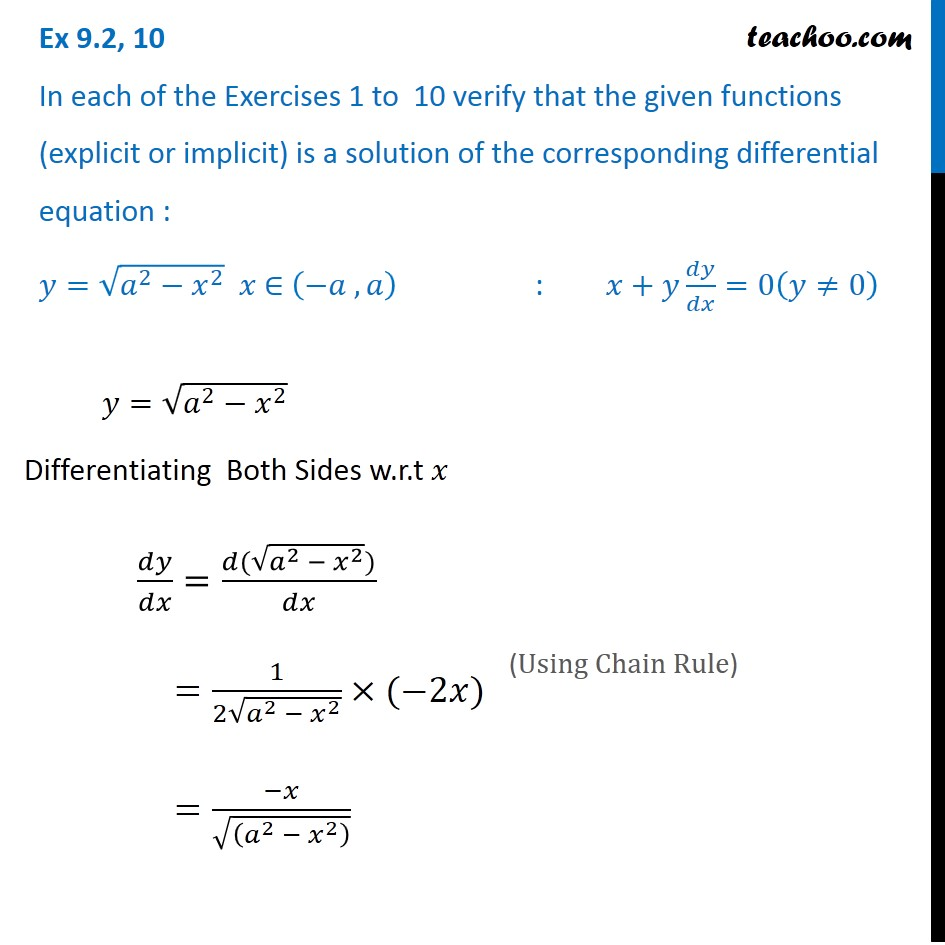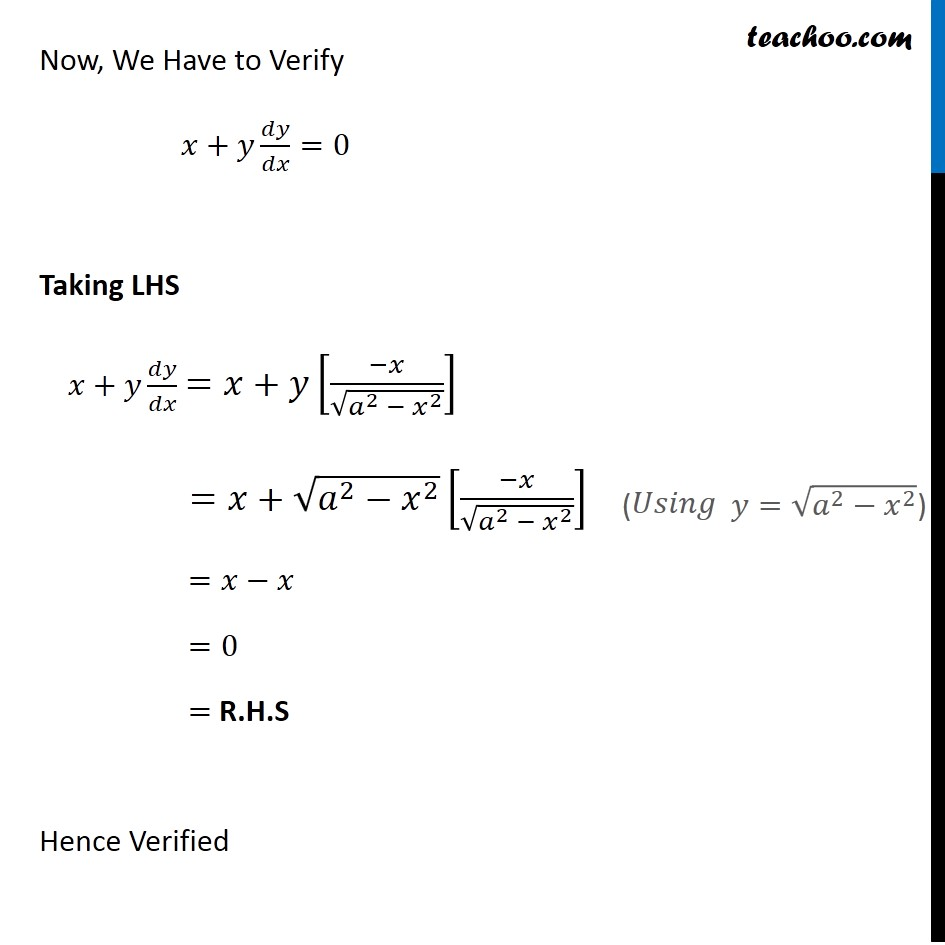Ex 9.2

Chapter 9 Class 12 Differential Equations
Serial order wiseLearn in your speed, with individual attention - Teachoo Maths 1-on-1 Class

### Transcript

Ex 9.2, 10 In each of the Exercises 1 to 10 verify that the given functions (explicit or implicit) is a solution of the corresponding differential equation : 𝑦=√(𝑎^2−𝑥^2 ) 𝑥 ∈ (−𝑎 , 𝑎) : 𝑥+𝑦 𝑑𝑦/𝑑𝑥=0(𝑦≠0) 𝑦=√(𝑎^2−𝑥^2 ) Differentiating Both Sides w.r.t 𝑥 𝑑𝑦/𝑑𝑥=(𝑑(√(𝑎^2 − 𝑥^2 )))/𝑑𝑥 =1/(2√(𝑎^2 − 𝑥^2 ))×(−2𝑥) =(−𝑥)/√((𝑎^2 − 𝑥^2 ) ) (Using Chain Rule) Now, We Have to Verify 𝑥+𝑦 𝑑𝑦/𝑑𝑥=0 Taking LHS 𝑥+𝑦 𝑑𝑦/𝑑𝑥 =𝑥+𝑦[(−𝑥)/√(𝑎^2 − 𝑥^2 )] =𝑥+√(𝑎^2−𝑥^2 ) [(−𝑥)/√(𝑎^2 − 𝑥^2 )] =𝑥−𝑥 =0 = R.H.S Hence Verified (█(𝑈𝑠𝑖𝑛𝑔 ) 𝑦=√(𝑎^2−𝑥^2 ))